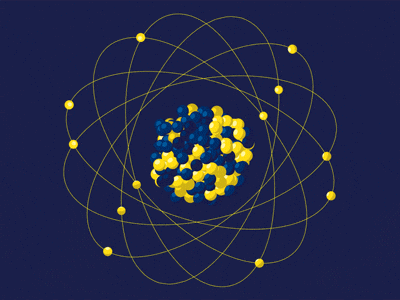Search

# Atomic&Ionic radii of group 1 and period 3Atomic radii - The measure of the disrance from the center of the nucleus to the boundary of the surrounding cloud of electrons orbiting it. Considering electrons do not have definite orbits, the atom is treates as a solid sphere instead, giving us an estimation instead.

Ionic radii (in a crystal lattice) - The measure of half the distance between two ions that are barely in contact. Considering electrons do not have definite orbits, the ion is treates as a solid sphere instead, giving us an estimation instead.

Atomic and Ionic radii of group 1 in the periodic tableThe atomic and ionic radii in group 1 increases and is very much so close to the trendline, considering down the group there is an increase in inner shell electrons, which means that there will be a decrease in effective nuclear charge and increase in the shielding effect, thus going down the group the elements will have an easier way of losing electrons.

Atomic and Ionic radii of Period 3 in the periodic tableThe atomic radii increases because there is an increase in effective nuclear charge, because of the increase of atomic numbers. The Ionic radii decreases, then increase and then decreases. It decreases first because there is a higher amount of protons than electrons, meaning an increase in effective nuclear charge which decreases its size, but then there is an increase between silicon and phosphorus because there is a new energy level and increase in electronegativity and as the effective nuclear charge increases there will be a major increase in ionic radii and then it decreases because because of the very effective nuclear charge and there is no increase in inner shell electrons so there is a decrease in ionic radii.